## Analyzing sequencing quality

I’ve been asked to analyze an exome sequencing trail where 100 samples were processed using ~20K probes and sequences using the Illumina platform. There are numerous things you can do, first using FastQC or other tools to analyze the average Phred score of reads, and maybe trim low quality bases off. But once that is done, there are numerous other things to look at.

This script revolves around some basic stast you can run to see what the overal alignment quality is of a large set of samples.

To do this we use sorted BAM files generated by aligning reads to a reference genome using BWA.

Running the bedtools genomecov with the following bash script will generate a list of each block in the reference with coverage >1, and the sum coverage that nucleotide has.

# This is the folder where all your files ending with .sorted.bam will be
FOLDER="/my_folder/*.sorted.bam"

# for each file in this FOLDER do an action
for file in $FOLDER do # To make the output a little nicer, we'll remove the directory FILE_NAME=${file##*my_folder/}

# Additionally we'll extract the actual sample name by removing additional naming information
# Our samples were names 1-PE-Ttru198.sorted.bam, 2-PE-Ttru198.sorted.bam, etc, so we can remove the tail so
SAMPLE_NAME=${FILE_NAME%\-PE-Ttru198.sorted.bam} # We can now run the genomecov on the${FILE_NAME} if you are IN the folder, or the ${FILE} if you are working # from another folder. The & character is used to push the process into the background so the loop can # innitate the next process, instead of waiting for it to finish first. bedtools genomecov -bga -split -ibam${FILE} > ${SAMPLE_NAME}_output_coverage.tsv & done wait$!

The [SAMPLE_NAME]_output_coverage.tsv files look something like this

##                  V1     V2     V3 V4
## 2 GeneScaffold_2815  66286  66356 28
## 1 GeneScaffold_2815  66230  66286 14
## 3 GeneScaffold_2815  66356  66410 14
## 4 GeneScaffold_2815 407074 407200  1
## 5 GeneScaffold_2815 955055 955178 10

Depending on the type of experiment you did, you can have reads aligning to your whole genome or just regions. Therefore the output file can become massive. This is why we can’t use Excell to check, but NEED R.

Additionally we also want just the number of reads per dataset, this helps to make some comparison between mapped and unmapped reads, usefull to find out if you have contamination or low quality sequencing sets.

Our set consists of paired end reads starting with SS_Task_1 and ending with .fastq.gz… you you can use this for single end or fasta as well

FILES="/mnt/d/Projects/SONATA-Stenella/ExomeData/*R1_001.fastq.gz"
for f in $FILES do FILE_NAME=${f##*ExomeData/SS_Task1_}
SAMPLE_NAME=${FILE_NAME%\_.*.fastq.gz} # Store the sample name echo -e -n${SAMPLE_NAME}'\t' >> sample_reads.txt
# Then store the reads... this one ONLY works on Fastq files that are ordered in 4 lines per read as it just counts
# the total lines and divides by 4
# Other options would be to use an additional 'zcat ${f} | grep ^@ | wc -l >>' for multi line fastq... or # 'zcat${f} | grep ^> | wc -l >>' for fasta files
zcat ${f} | echo$((wc -l/4)) >> sample_reads.txt
done

The sample_reads.txt files look something like this

##   Name   Reads
## 1   10  518775
## 2   11 1137841
## 3   13 5125203
## 4   14 1175029
## 5   15 1428862

Now that we have our data generated, we can run some analysis in R. My R scripts always include these cleanup functions to attatch and detachs packages as plyr and dplyr are usefull packages but incompattable with each other.

# A random function found on stackoverflow to remove all currently loaded pacakges... need this to prevent plyr/dplyr conflicts
detachAllPackages <- function() {
basic.packages <- c("package:stats","package:graphics","package:grDevices","package:utils","package:datasets","package:methods","package:base")
package.list <- search()[ifelse(unlist(gregexpr("package:",search()))==1,TRUE,FALSE)]
package.list <- setdiff(package.list,basic.packages)
if (length(package.list)>0)  for (package in package.list) detach(package, character.only=TRUE)
}

#Function to check if package is already installed, if not, installs it. After install it and loads it
for(package in Required_Packages){
if (!package %in% installed.packages()) install.packages(package, character.only = TRUE)
library(package, character.only = TRUE)
}
}

detaching packages is really simple with this detachAllPackages() function, and the install_load() evaluates whether something is already installed or not, if not it will install it, and if it is already installed it will just load it. And the usefull thing is you can just give it a list of packages and let it do the work for you

Next we want to load our files… personally i like having an interface so i load folders with choose.dir() and load individual files with file.choose(). But you could also just make a hard-coded path for these.

The folder containing our [SAMPLE_NAME]_output_coverage.tsv is stored in files_list, and our single sample_reads.txt is loaded in read_file.

#Load a folder of files generated with
files_list <- list.files(path=choose.dir(), pattern="*.tsv", full.names=TRUE, recursive=FALSE)
read_file <- read.csv(file.choose(), sep="\t", na.strings=c("","NA"), header = FALSE)

We also need to make to blank datatables as we are going to loop through the coverage.tsv files and store it to a pre defined variable.

coverage_distro <- data.frame(Name=character(),
Coverage =character(),
Frequency=character(),
stringsAsFactors=TRUE)

average_coverage_data <- data.frame(Name=character(),
Ref_nuc_span=character(),
Samp_nucs_mapped=character(),
Average_coverage=character(),
stringsAsFactors=TRUE) 

The naming here was meant to be intuitive but essentially we’ll end up with a data.frame containing a distribution of coverage (how many nucleotides have x coverage) and a data.frame that holds summary data for each sample (how many reference nucleotides have something mapped to them, how many read nucleotides are mapped in total, what is the average coverage, etc)

We’ll load the plyr package, as we’ll use ddplyr a little later on, and initiate the loop

# Install and load the following packages
for (file in files_list) {
# Load one sample file at a time
# The only column we'll keep is covverage, so we'll lable this one to make it easier
colnames(table_input) <- "Coverage"
# Extract sample name
sample_name = as.numeric(sub("_coverage.tsv","",sub(".*\\\\","",file)))
# Column bind, sample name, the coverage value, and we'll also calculate the length of the nucleotide block
# generated because we used the -bga function in genomecov. If you have a small genome you can use -d
table_input$Name <- sample_name table_input$Ref_nuc_span <- c(table_input[,3]-table_input[,2])
table_input <- table_input[,-c(1:3)]
#Remove all lines with zero coverage... you can also prefilter these
table_input <- table_input[which(table_input$Coverage!=0),] # Add column containing multiplecation of coverage times nucleotides in block to get total nucleotides derived # from sample reads, mapped over that area table_input$Samp_nucs_mapped <- c(table_input$Coverage*table_input$Ref_nuc_span)
# Using ddply, we can sum the amount of reference nucleotides that have the same Coverage, so we can generate a
# Coverage per nucleotide matrix for each sample. And we'll append it to Coverage_distro for later use
temp <- cbind(sample_name,ddply(table_input, .(Coverage), function(x) sum(x[,3])))
colnames(temp) <- c("Name","Coverage","Frequency")
coverage_distro <- rbind(coverage_distro,temp)

# Make a new table containing the name, total reference nucleotides covered, total sample nucleotides
# aligned and average coverage
coverage_data <- data.frame(Name = sample_name,
Ref_nuc_span = sum(table_input[,"Ref_nuc_span"]),
Samp_nucs_mapped = sum(table_input[,"Samp_nucs_mapped"]),
Average_coverage = sum(table_input[,"Samp_nucs_mapped"])/
sum(table_input[,"Ref_nuc_span"]))

# Join the coverage_Data to the average_coverage_data table, this will be our overview table for each sample
average_coverage_data <- rbind(average_coverage_data,coverage_data)

}

Cool, so now we have 2 data.frames. The first contains a distribution of nucleotides per coverage for each sample. The second contain as general overview of reads mapped, reference nucleotides covered etc.

Now we have some basic summary information per sample in our average_coverage_data data.frame, that looks like this.

##   Name Ref_nuc_span Samp_nucs_mapped Average_coverage
## 1   10      7276699        116633066        16.028293
## 2   11     43351306        234853371         5.417446
## 3   13    151620149        653200678         4.308139
## 4   14     12409859        213832370        17.230846
## 5   15     39723600        268541906         6.760261

Now that this is done, we don’t need plyr anymore so we can detach it, and we’ll need some other tools including dplyr and some tools for visualization

detachAllPackages()
"dplyr",
"cowplot",
"viridis"))

We opened the sample_reads.txt before but haven’t used it yet. But now that we have the summary data.frame, we can easily add the reads to that overview like so.

# Add a column of Reads that we obtained seperately to the average_coverage_data file
# In our example, reads are paired end, but we only calculated reads in R1, not R2, so we'll just multiply by 2
read_file$Reads <- c(read_file$Reads * 2)
# And now we can merge our reads per sample with our sample summary data
average_coverage_data2 <- merge(average_coverage_data, read_file, by.x="Name", by.y="Name", sort = TRUE)

As we are planning to plot these data.frames using some boxplots, it’s usefull to add some reference lines that indiciate what the mean value per datatype is.

# We have several count data now in our average_coverage_data2 data.frame. But in a graph it's nice to see what the
# mean is of all samples so you can see which are higher, which is lower, and if the average is even enough for the
# purpose of your study.So we'll generate the mean and later use it to plot a mean line on the graph.
average_coverage_data2 <- average_coverage_data2 %>% mutate(Mean_ref_nuc_span = mean(Ref_nuc_span))
average_coverage_data2 <- average_coverage_data2 %>% mutate(Mean_tot_nucs_aligned = mean(Samp_nucs_mapped))

# Additionally for the coverage data, it's often suggested to have a coverage between 10x and 30x, but this can be
# different depending on the project. But let's just go ahead and add those values as lines so whe know
# which samples had an average coverage that at least matches.
average_coverage_data2 <- average_coverage_data2%>%  mutate(mean_cov = mean(Average_coverage))
average_coverage_data2$tenx = 10 average_coverage_data2$thirtyx = 30

# Lastly, the mean reads, used to generate the indicater line later on
average_coverage_data2 = average_coverage_data2 %>% mutate(mean_rd = mean(Reads))

Because we have calcultated the # of sample nucleotides mapped to the reference, and we know the number of reads per sample, we can also estimate the amount of unmapped nucleotides by just subtracting the mapped from the total estimate.

# As we'll exploit the aes fill option to produce our seperate barplots for each type, we'll have to make some
# temporary data.frames, with different identifiers, in this case $label, so we can use it later. average_coverage_tmp1 <- average_coverage_data2 %>% mutate(Mean_Samp_nucs_mapped = mean(Samp_nucs_mapped)) average_coverage_tmp1$label <- "Mapped"
average_coverage_tmp2 <- average_coverage_tmp1 %>% mutate(Samp_nucs_mapped = Reads*100)
average_coverage_tmp2$label <- "Est. Total" average_coverage_tmp3 <- average_coverage_tmp1 %>% mutate(Samp_nucs_mapped = c((Reads*100) - Samp_nucs_mapped )) average_coverage_tmp3$label <- "Unmapped"
average_coverage_tmp <- rbind(average_coverage_tmp1,average_coverage_tmp2,average_coverage_tmp3)

We now have everything needed to start plotting. Firstly let’s plot the nucleotides per coverage plots for each sample. This will be a big figure if you have a lot of samples, so make sure you adjust the width and height of the output pdf accordingly.

colnames(coverage_distro) <- c("Name","Coverage","Frequency")
# The first plot generates the coverage distribution per sample, so these will be 100 little plots in one
pdf("Coverage_distributions.pdf", width=18,height=14)
p1 <-   ggplot(coverage_distro, aes(x=Coverage, y=Frequency, group=Name, fill=Name)) +
geom_area() +
# Sometimes there is an outlier datapoint, in this case we have a small tail of 1 nucleotide having 450x
# coverage. These points don't add much, so we'll just look at the 0-30x coverage region
scale_x_continuous(limit=c(0,30)) +
theme(legend.position="none") +
theme(legend.position="none",
panel.spacing = unit(0.1, "lines"),
strip.text.x = element_text(size = 8)) +
#Make a plot for each unique identifrier found in \$Name
facet_wrap(~Name, scale="free_y")
p1
dev.off()
## Warning: Removed 71781 rows containing missing values (position_stack).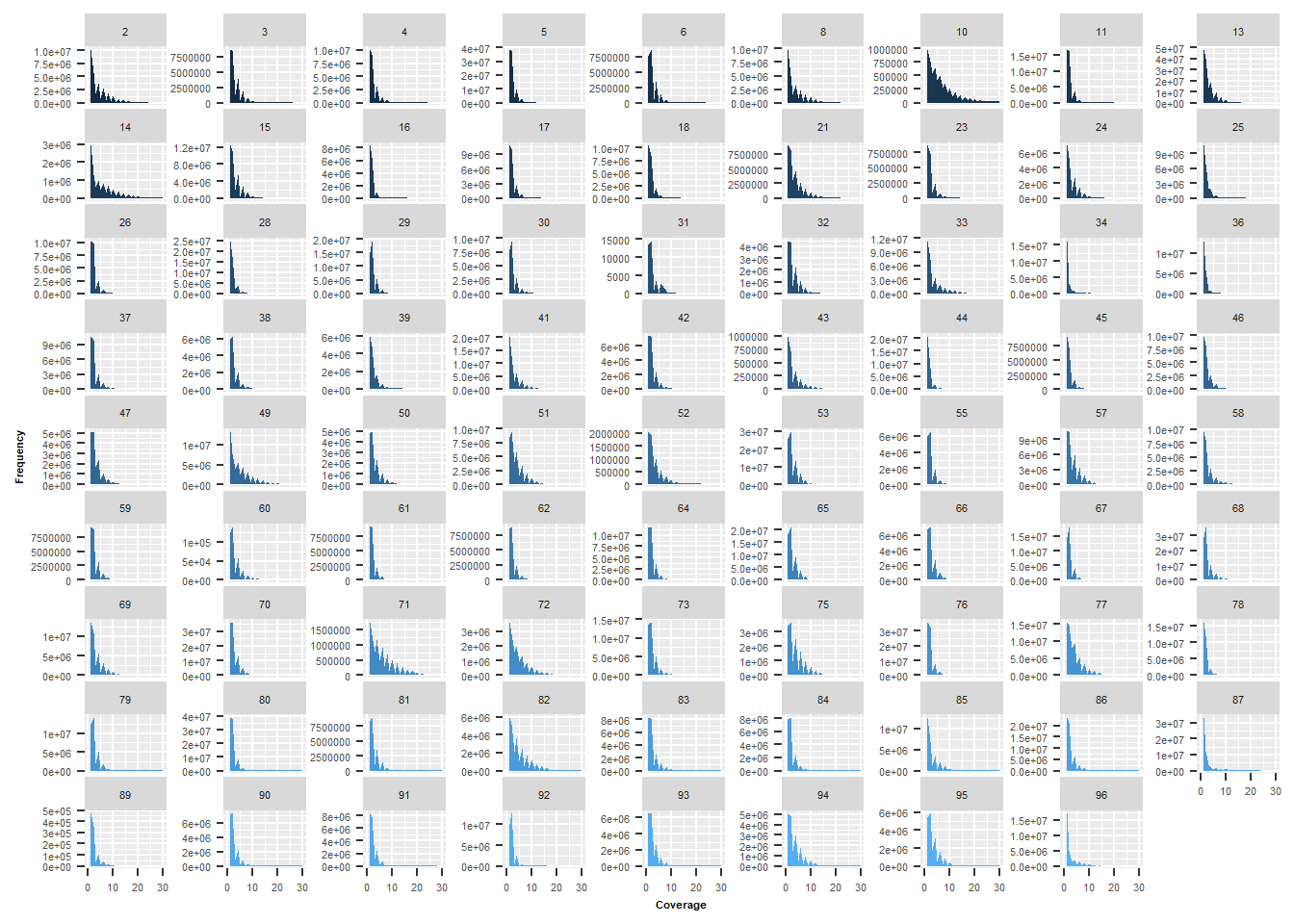As mentioned before, the figure is huge, but compressed in a little webpage it gets unreadable which is why you probably have to change the length and width of your document based on . But it’s a good figure to observe a trend in your data. For example a lot of our data has a peak around 2-6x coverage, well below 10x. This indicates that the sequencing depth is not good enough. Several samples also show that the coverage is only found in ~1000 nucleotides maximum. As we are trying to span a 3.5Mb exone region, this shows that there might be a problem with mapping.

The following plots will help figure out what that problem could be. The first plot will show how many reference genome nucleotides have a coverage of 1 or higher.

pdf("Sample_read_quality.pdf", width=18,height=12)
plot1 <-  average_coverage_data2 %>%
select(Name, Ref_nuc_span ) %>%
na.omit() %>%
ggplot() +
geom_bar(aes(x = Name, y = Ref_nuc_span ), stat = "identity", alpha = 0.75, fill = "palegreen3") +
ylab("Chrom Nuc covered") +
# We'll use the previously calculated mean value to generate a dotted line
geom_errorbar(data=average_coverage_data2, aes(Name, ymax = Mean_ref_nuc_span, ymin = Mean_ref_nuc_span),
size=0.5, linetype = "longdash", inherit.aes = F, width = 1) +
theme_minimal() +
# As we'll we'll be merging all plot into one nice pdf at the end, we'll ommit all x-labels
theme(axis.title.x = element_blank(),
axis.text.x = element_blank())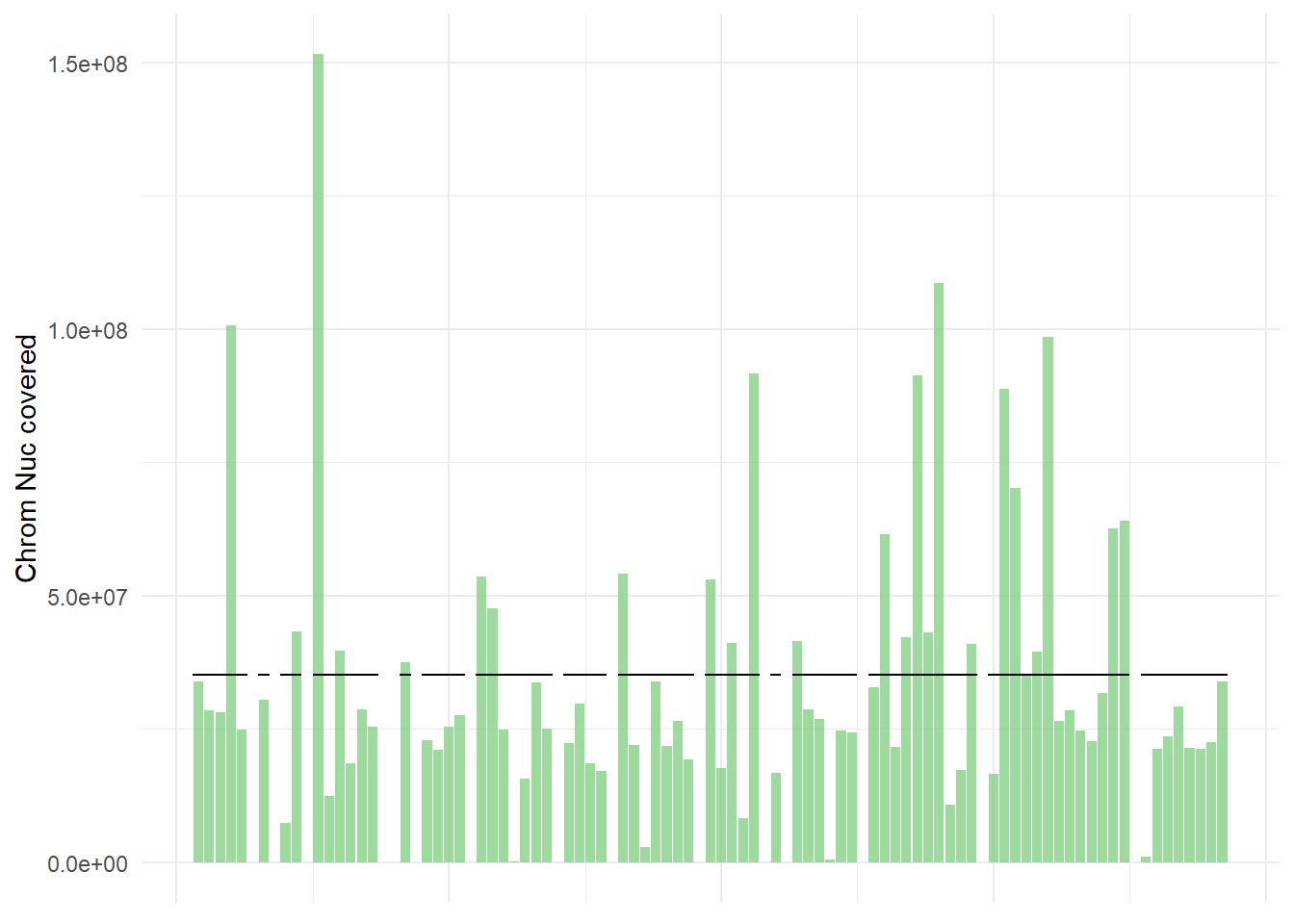The second plot will show the mapped nucleotides, total available nucleotides (based on reads * 100 in size) and the unmapped nucleotides (total – mapped).

plot2 <-  average_coverage_tmp %>%
select(Name, Samp_nucs_mapped,label) %>%
na.omit() %>%
ggplot() +
geom_bar(aes(x = Name, y = Samp_nucs_mapped , fill=label), stat = "identity", position=position_dodge()) +
# We again will add a dotted means line
geom_errorbar(data=average_coverage_tmp, aes(Name, ymax = Mean_Samp_nucs_mapped, ymin = Mean_Samp_nucs_mapped),
size=0.5, linetype = "longdash", inherit.aes = F, width = 1) +
scale_fill_brewer(palette="Accent")+
theme_minimal() +
# We do want a legend, but we want to make it as small as possible
guides(shape = guide_legend(override.aes = list(size = 100)),
color = guide_legend(override.aes = list(size = 100))) +
theme(axis.title.x = element_blank(),
axis.text.x = element_blank(),
#        legend.position = "none")
legend.title = element_blank(),
legend.text=element_text(size=4),
legend.position = c(0.95, 0.8))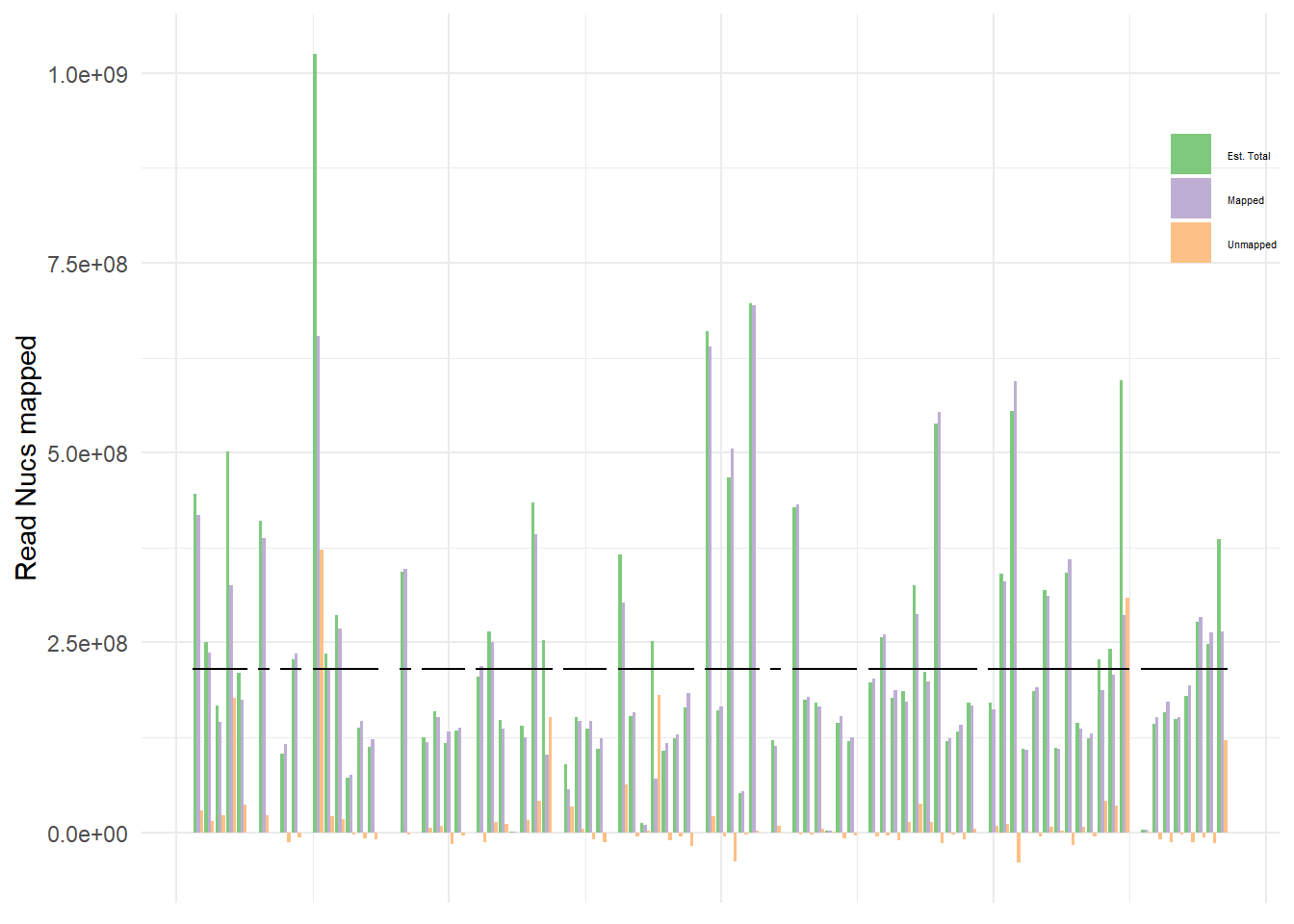The third plot is the average coverage observed.

# Instead of 1 trendline, we'll add 3, one to highlight where 10x is, one where 30x is and the mean value
# of all samples. For this we'll use predefined lables and colors.
cols <- c("Mean"="black","10X"="maroon","30X"="seagreen1")
plot3 <-  average_coverage_data2 %>%
select(Name, Average_coverage) %>%
na.omit() %>%
ggplot() +
geom_bar(aes(x = Name, y = Average_coverage), stat = "identity", alpha = 0.75, fill = "slategray3") +
ylab("Avg coverage") +
# Here we'll add our dotted lines, using the predefined colors
geom_errorbar(data=average_coverage_data2, aes(Name, ymax = mean_cov, ymin = mean_cov, colour="Mean"),
size=0.5, linetype = "longdash", inherit.aes = F, width = 1) +
geom_errorbar(data=average_coverage_data2, aes(Name, ymax = tenx, ymin = tenx, colour="10X"),
size=0.5, linetype = "longdash", inherit.aes = F, width = 1) +
geom_errorbar(data=average_coverage_data2, aes(Name, ymax = thirtyx, ymin = thirtyx, colour="30X"),
size=0.5, linetype = "longdash", inherit.aes = F, width = 1) +
theme_minimal() +
# We again want a very tiny legend that fit inside the plot, so we can easily align plots later
scale_colour_manual(name="Error Bars",values=cols) +
scale_fill_manual(name="Bar",values=cols) +
theme(axis.title.x = element_blank(),
axis.text.x = element_blank(),
legend.title = element_blank(),
legend.text=element_text(size=4),
legend.position = c(0.90, 0.8))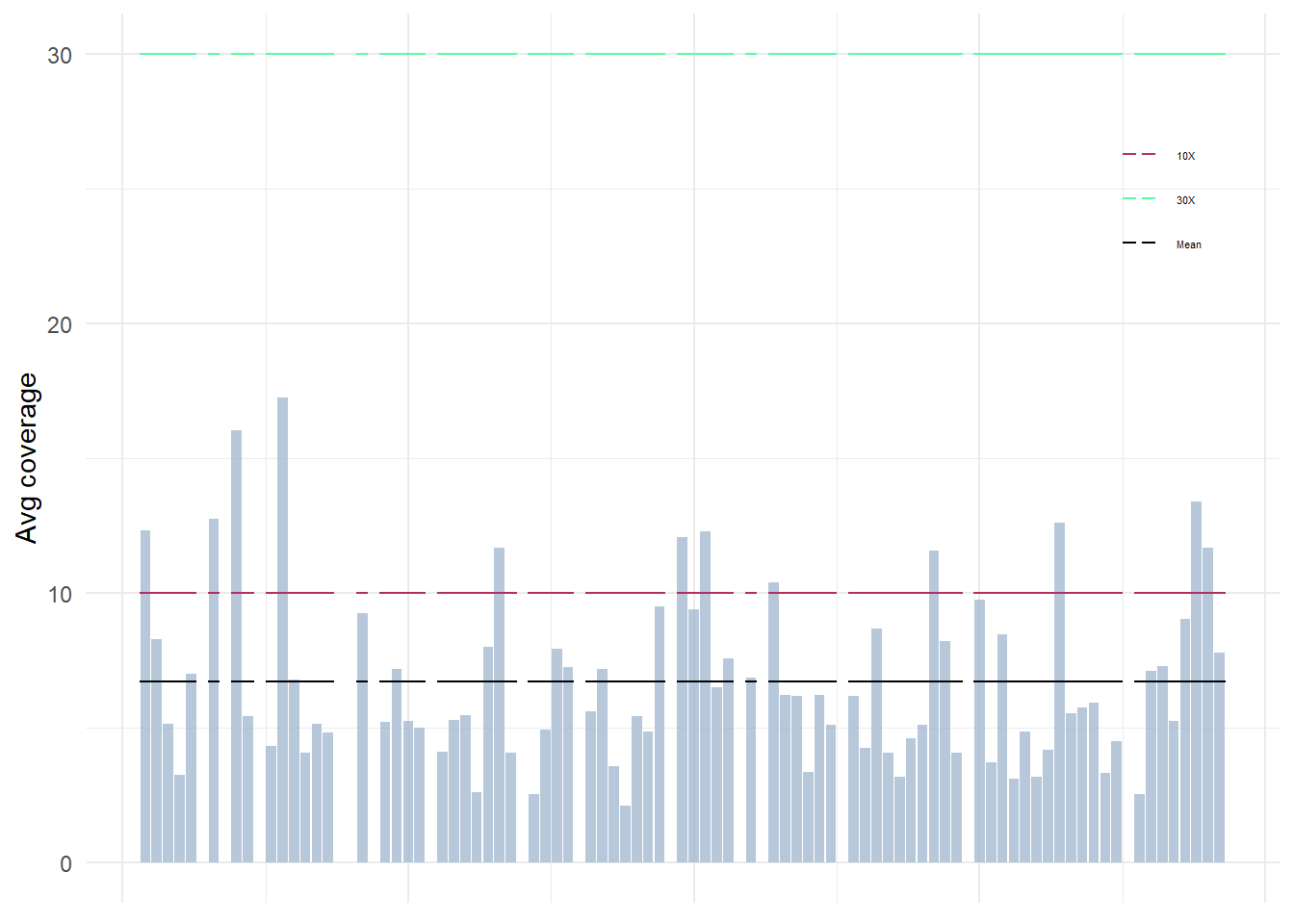The last plot is just to show the amount of reads per sample. if you want you can combine plot2 and plot4 into one plot as essentially the total amount of sample nucleotides is the exact same thing as total amount of reads, just a factor 100 off. You can either devide the number of mapped nucleotides by 100… or convert it into a percentage. Many ways to go about it. For this case i left it seperate to just focus on samples with very low sequencing quality.

plot4 <-  average_coverage_data2 %>%
na.omit() %>%
ggplot() +
geom_bar(aes(x = Name, y = Reads), stat = "identity", alpha = 0.75, fill = "palevioletred2") +
geom_errorbar(data=average_coverage_data2, aes(Name, ymax = mean_rd, ymin = mean_rd),
size=0.5, linetype = "longdash", inherit.aes = F, width = 1) +
theme_minimal() +
theme(axis.title.x = element_blank())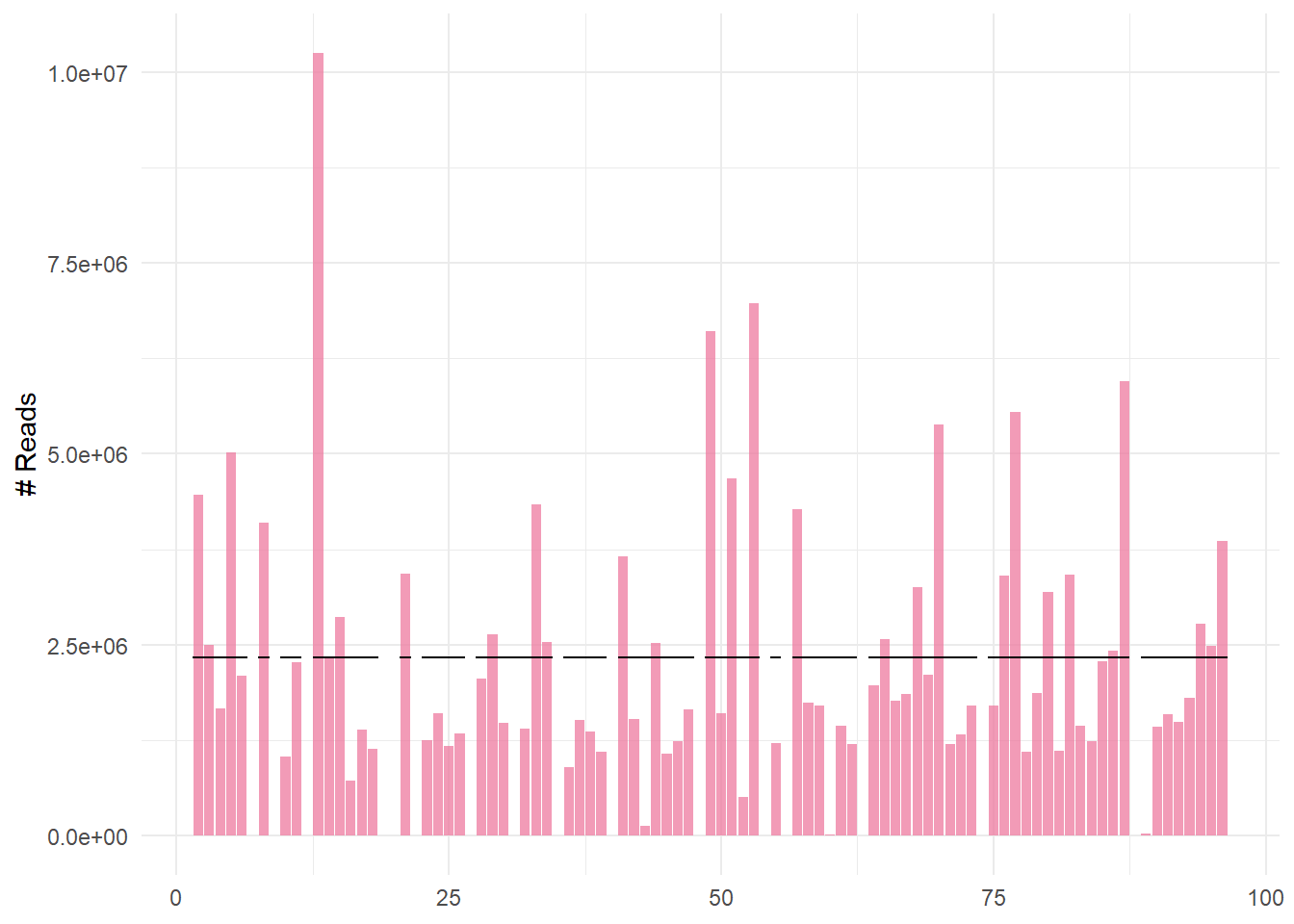Finally we can plot all the 4 plots on top of each other and bask in the glory of R visualizations

plot_grid(plot1, plot2, plot3, plot4, align = "v", ncol = 1, rel_heights = c(0.24, 0.24, 0.24, 0.28))
dev.off()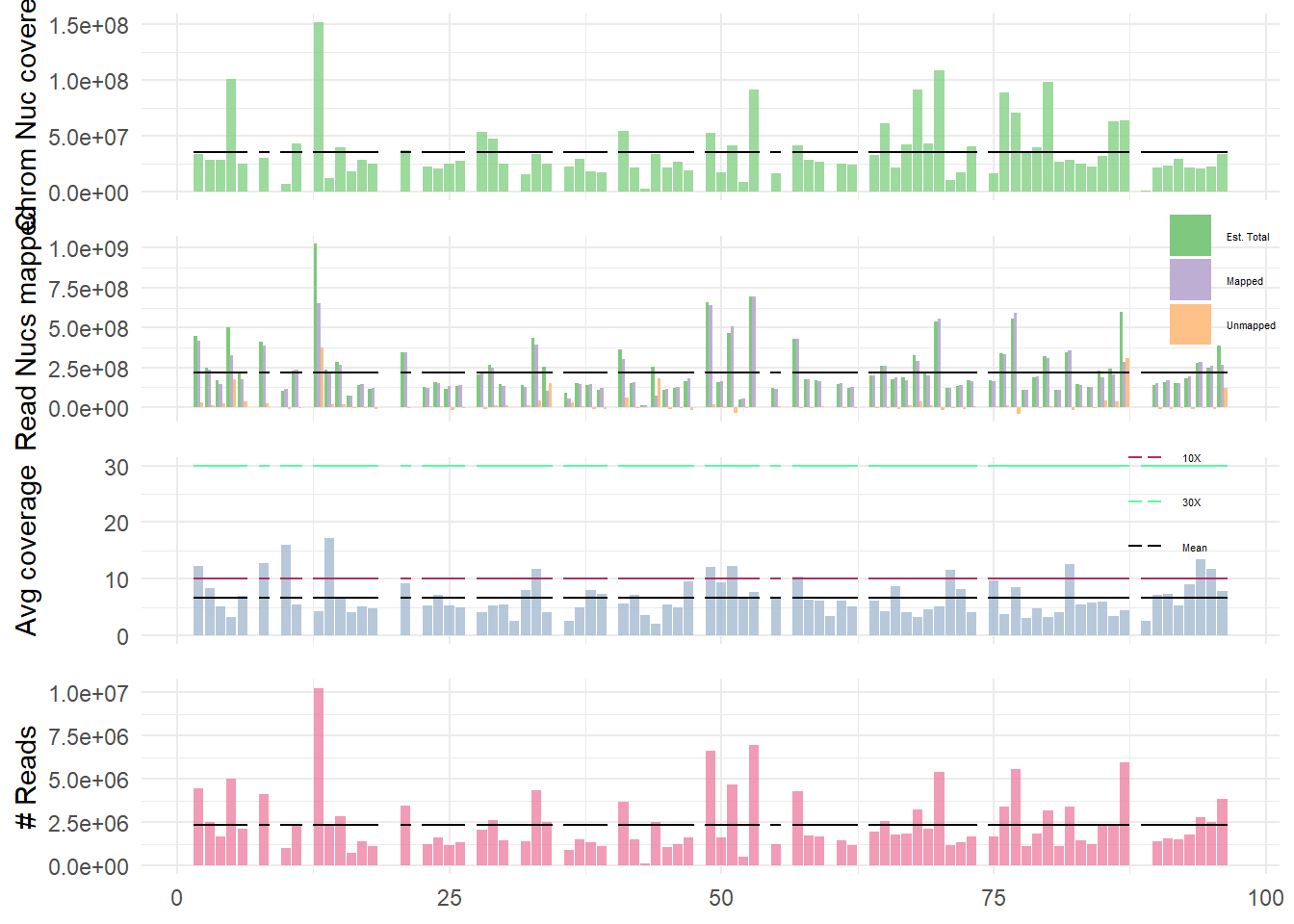And that is it… you can now easily interpret, which samples have poor sequencing based on reads. Whic have poor coverage (and potentially contamination if the reads are high, but coverage low). You can deduce if there is maybe some issue with your alignment software by looking at mapped vs. unmapped read nucleotides. And you can look at how well your reference genome is covered (this could be used to find out if samples of distant species are likelyto lacking genetic region. This all before even looking at any of the alignments.

Thanks for flying Lesley airlines, till next time